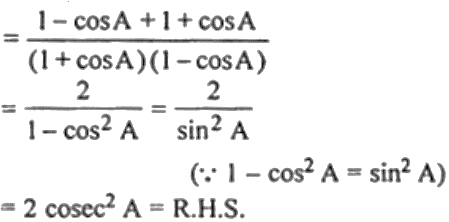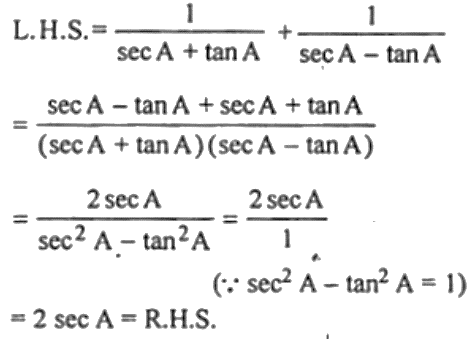Guru

# (i) 1/ (1 + cos A) + 1/ (1 – cos A) = 2 cosec2 A (ii) 1/(sec A + tan A) + 1/(sec A – tan A) = 2 sec A

• 0

This question has been taken form the

Book- ML Aggarwal
Board- ICSE
Publication- Avichal
Chapter- Trigonometric Identities
Chapter number-18

We have to prove that

(i) 1/ (1 + cos A) + 1/ (1 – cos A) = 2 cosec2 A

(ii) 1/(sec A + tan A) + 1/(sec A – tan A) = 2 sec A

Class10th. Trigonometric Identities, ques nom 14

Share